# Search

About 245 Search Results Matching Types of Worksheet, Worksheet Section, Generator, Generator Section, Subjects matching Math, Grades matching 3rd Grade, Similar to Reading a Protractor Worksheet 2, Page 2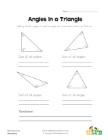## Angles in a Triangle Worksheet

Kids learn that the angles of a triangle always ad...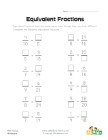## Equivalent Fractions Worksheet 2

Fill in the missing numbers to make equivalent fra...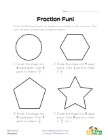## Fraction Fun Worksheet 2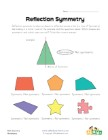## Symmetry Worksheet 2

Which shapes are symmetric and which are not?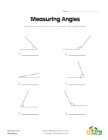## Measuring Angles Worksheet 1

Measure each of the angles with a protractor and w...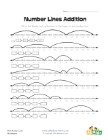## Number Lines Addition Worksheet 2

Fill in the blanks in the equations by using the n...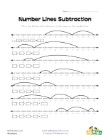## Number Lines Subtraction Worksheet 2

Fill in the blanks in the equations by using the n...